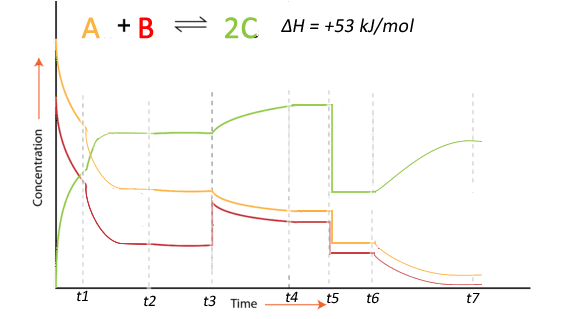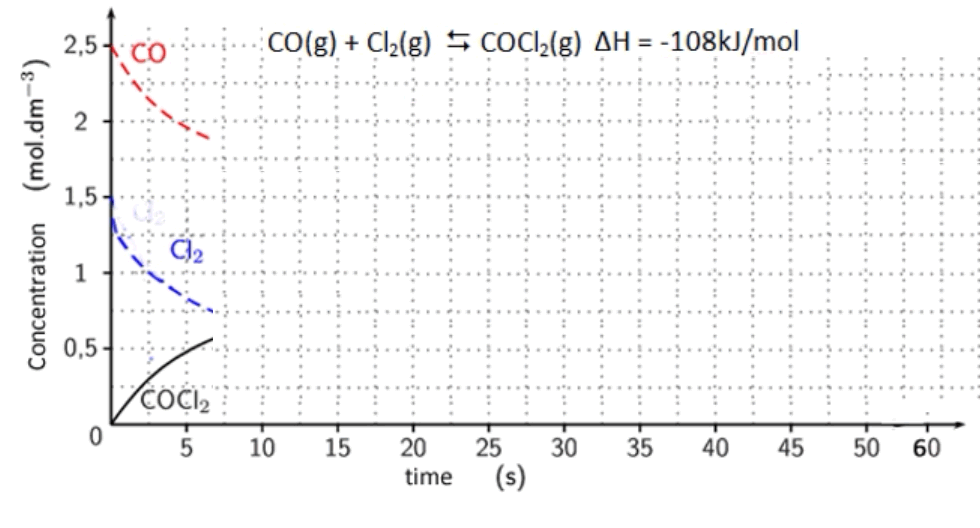Equilibrium Concentration time graphs Consider the equilibrium system shown on the right. A number of stresses have been applied and the response recorded in the concentration vs time graph shown on the right. 1. What is the best explanation for the likely stress applied to the system at t1 2. Compare the value of the reaction quotient (Qc ) between t2 and t3  and the reaction quotient between t4 and t5. 3. What was the likely stress applied to the system at t3  4. What was the likely stress applied to the system at t5 5. What was the likely stress applied to the system at t6 6. Compare the value of the reaction quotient (Qc ) at t7  and the reaction quotient at t4.Refer to the video for solutions 3. Consider the equilibrium system shown on the right. Click to download a word document with the concentration vs time graph shown on the right. Print this document and complete the graph indicating the following stresses and the response from the system. a. The system reaches equilibrium withing 12 seconds. b. Then the following stresses are applied.     i.    CO is added and its concentration increases to 2.2M     ii.    Equilibrium is re-established within 5 seconds.              Where at the 35 second mark the temperature is            reduced and equilibrium is reached within 5 seconds     iii.   At 40 seconds a catalyst is added.     iv.   At 45 seconds COCl2 is added.      v.   The volume of the reaction vessel is increased by            10%.View the video on the right for solutions